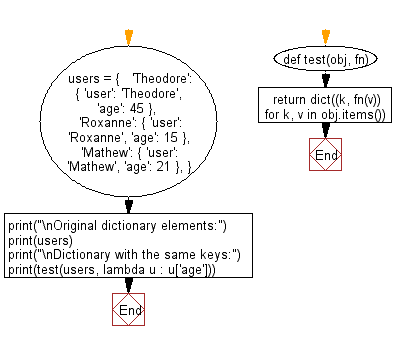﻿ Python: Creates a dictionary with the same keys as the given dictionary and values generated by running the given function for each value - w3resource# Python: Creates a dictionary with the same keys as the given dictionary and values generated by running the given function for each value

## Python dictionary: Exercise-74 with Solution

Write a Python program to create a dictionary with the same keys as the given dictionary and values generated by running the given function for each value.

Sample Solution:

Python Code:

``````def test(obj, fn):
return dict((k, fn(v)) for k, v in obj.items())

users = {
'Theodore': { 'user': 'Theodore', 'age': 45 },
'Roxanne': { 'user': 'Roxanne', 'age': 15 },
'Mathew': { 'user': 'Mathew', 'age': 21 },
}
print("\nOriginal dictionary elements:")
print(users)
print("\nDictionary with the same keys:")
print(test(users, lambda u : u['age']))
```
```

Sample Output:

```Original dictionary elements:
{'Theodore': {'user': 'Theodore', 'age': 45}, 'Roxanne': {'user': 'Roxanne', 'age': 15}, 'Mathew': {'user': 'Mathew', 'age': 21}}

Dictionary with the same keys:
{'Theodore': 45, 'Roxanne': 15, 'Mathew': 21}
```

Flowchart:## Visualize Python code execution:

The following tool visualize what the computer is doing step-by-step as it executes the said program:

Python Code Editor:

Have another way to solve this solution? Contribute your code (and comments) through Disqus.

What is the difficulty level of this exercise?

Test your Programming skills with w3resource's quiz.

﻿

## Python: Tips of the Day

Decapitalizes the first letter of a string:

Example:

```def tips_decapitalize(s, upper_rest=False):
return s[:1].lower() + (s[1:].upper() if upper_rest else s[1:])
print(tips_decapitalize('PythonTips'))
print(tips_decapitalize('PythonTips', True))
```

Output:

```pythonTips
pYTHONTIPS
```